Graphics Output

This section describes the use of ODS for creating graphics with the SEQTEST procedure. To request these graphs, ODS Graphics must be enabled and you must specify the associated graphics options in the PROC SEQTEST statement. For more information about ODS Graphics, see Chapter 21, Statistical Graphics Using ODS.

### Sequential ASN Plot

The PLOTS=ASN option displays the average sample numbers (expected sample sizes for nonsurvival data or expected numbers of events for survival data) under various hypothetical references. The average sample numbers are connected for each design, and these connected curves for all designs are displayed in the "Sequential ASN Plot" graph.

For a one-sided design, average sample numbers under the hypothetical references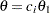are displayed, where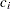are the values specified in the CREF= option and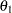is the alternative reference. The horizontal axis displays thevalues of these hypothetical references.

For a two-sided design, average sample numbers under each of the hypothetical referencesand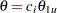are displayed, where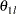andare the lower and upper alternative references, respectively. The horizontal axis displays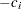values for lower hypothetical referencesandvalues for upper hypothetical references. Note that for a symmetric two-sided design, only average sample numbers under the hypothetical referencesare derived.

If the trial stops after the sequential test, the hypothetical reference corresponding to the test statistic is also indicated in the plot.

### Conditional Power Plot

The PLOTS=CONDPOWER option displays the conditional powers given the observed statistic under various hypothetical references. These powers are connected and are displayed in the "Conditional Power Plot" graph.

For a one-sided test, the power are derived under the hypothetical references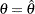and, where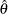is the observed statistic,is the alternative reference, andare the values specified in the CREF= option. The horizontal axis displays thesevalues for hypothetical references.

For a two-sided test, the power are derived under hypothetical references,, and, whereis the lower alternative reference andis the upper alternative reference. The horizontal axis displaysvalues for hypothetical referencesandvalues for hypothetical references.

If the trial stops after the sequential test, the hypothetical reference corresponding to the test statistic is also indicated in the plot.

### Sequential Error Spending Plot

The PLOTS=ERRSPEND option displays the cumulative error spending at each stage on each boundary in the "Sequential Error Spending Plot" graph. A legend table uses the design labels to identify the curves for the corresponding design in the plot.

### Sequential Power Plot

The PLOTS=POWER option displays the powers under various hypothetical references. The powers are connected for each design, and these connected curves for all designs are displayed in the "Sequential Power Plot" graph.

For a one-sided design, powers under hypothetical referencesare displayed, whereare the values specified in the CREF= option andis the alternative reference. The horizontal axis displays thevalues of these hypothetical references.

For a two-sided design, powers under hypothetical referencesandare displayed, whereandare the lower and upper alternative references, respectively. The horizontal axis displaysvalues for lower hypothetical referencesandvalues for upper hypothetical references. Note that for a symmetric two-sided design, only powers under hypothetical referencesare derived.

If the trial stops after the sequential test, the hypothetical reference corresponding to the test statistic is also indicated in the plot.

### Repeated Confidence Intervals Plot

The PLOTS=RCI option displays repeated confidence intervals at each stage given the observed statistic at that stage. These repeated confidence intervals are displayed in the "Repeated Confidence Intervals Plot" graph.

### Sequential Test Plot

The PLOTS=TEST option displays boundary values and test statistics in the "Test Plot" graph. The boundary values are connected for each boundary, and both the stage number and the information level at each stage are displayed. The legend table identifies the acceptance and rejection regions in the plot.